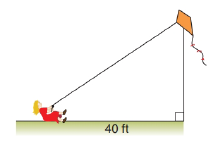Chapter 5.4, Problem 16EElementary Geometry For College St...

7th Edition
Alexander + 2 others
ISBN: 9781337614085

Solutions

Chapter
SectionElementary Geometry For College St...

7th Edition
Alexander + 2 others
ISBN: 9781337614085
Textbook Problem

A strong wind holds a kite 30 ft above the earth in a position 40 ft across the ground. How much string does the girl let out to the kite?To determine

To Find:

Length of the string is 50ft.

Explanation

Given A strong wind holds a kite 30 ft above the earth in a position 40 ft across the ground.

Let x be the length of the string.

From the figure it is in form of right triangle in which both the sides are 30 ft. and 40 ft.

From the given data,

x2=302+

Still sussing out bartleby?

Check out a sample textbook solution.

See a sample solution

The Solution to Your Study Problems

Bartleby provides explanations to thousands of textbook problems written by our experts, many with advanced degrees!

Get Started

In Exercises 1-6, simplify the expression. (20)2+[ 7(3) ]2

Calculus: An Applied Approach (MindTap Course List)

Let f(x) = 2x2 x + 1. Find: a. f(x 1) + f(x + 1) b. f(x + 2h)

Applied Calculus for the Managerial, Life, and Social Sciences: A Brief Approach

Calculate y'. 10. y = emx' cos nx

Single Variable Calculus: Early Transcendentals, Volume I

Evaluate the expression sin Exercises 116. 23

Finite Mathematics and Applied Calculus (MindTap Course List)

Simplify each power of i. i33

Trigonometry (MindTap Course List)

The distance between (7, 4, –3) and (–1, 2, 3) is: 4 16 104

Study Guide for Stewart's Multivariable Calculus, 8th

For y = 3x + tan x, y = _____. a) 3 + sec x b) 3x sec2 x + 3 tan x c) 3 + sec2 x d) x cos x + sin x

Study Guide for Stewart's Single Variable Calculus: Early Transcendentals, 8th